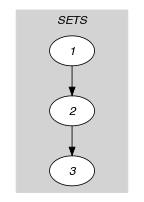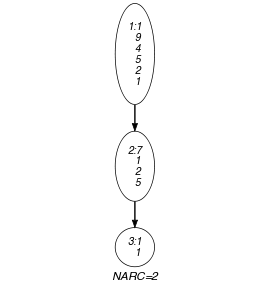## 5.213. k_used_by_modulo

Origin
Constraint

$𝚔_\mathrm{𝚞𝚜𝚎𝚍}_\mathrm{𝚋𝚢}_\mathrm{𝚖𝚘𝚍𝚞𝚕𝚘}\left(\mathrm{𝚂𝙴𝚃𝚂},𝙼\right)$

Type
 $\mathrm{𝚅𝙰𝚁𝙸𝙰𝙱𝙻𝙴𝚂}$ $\mathrm{𝚌𝚘𝚕𝚕𝚎𝚌𝚝𝚒𝚘𝚗}\left(\mathrm{𝚟𝚊𝚛}-\mathrm{𝚍𝚟𝚊𝚛}\right)$
Arguments
 $\mathrm{𝚂𝙴𝚃𝚂}$ $\mathrm{𝚌𝚘𝚕𝚕𝚎𝚌𝚝𝚒𝚘𝚗}\left(\mathrm{𝚜𝚎𝚝}-\mathrm{𝚅𝙰𝚁𝙸𝙰𝙱𝙻𝙴𝚂}\right)$ $𝙼$ $\mathrm{𝚒𝚗𝚝}$
Restrictions
 $\mathrm{𝚛𝚎𝚚𝚞𝚒𝚛𝚎𝚍}$$\left(\mathrm{𝚅𝙰𝚁𝙸𝙰𝙱𝙻𝙴𝚂},\mathrm{𝚟𝚊𝚛}\right)$ $|\mathrm{𝚅𝙰𝚁𝙸𝙰𝙱𝙻𝙴𝚂}|\ge 1$ $\mathrm{𝚛𝚎𝚚𝚞𝚒𝚛𝚎𝚍}$$\left(\mathrm{𝚂𝙴𝚃𝚂},\mathrm{𝚜𝚎𝚝}\right)$ $|\mathrm{𝚂𝙴𝚃𝚂}|>1$ $\mathrm{𝚗𝚘𝚗}_\mathrm{𝚒𝚗𝚌𝚛𝚎𝚊𝚜𝚒𝚗𝚐}_\mathrm{𝚜𝚒𝚣𝚎}$$\left(\mathrm{𝚂𝙴𝚃𝚂},\mathrm{𝚜𝚎𝚝}\right)$ $𝙼>0$
Purpose

Given $|\mathrm{𝚂𝙴𝚃𝚂}|$ sets of domain variables, the $𝚔_\mathrm{𝚞𝚜𝚎𝚍}_\mathrm{𝚋𝚢}_\mathrm{𝚖𝚘𝚍𝚞𝚕𝚘}$ constraint forces a $\mathrm{𝚞𝚜𝚎𝚍}_\mathrm{𝚋𝚢}_\mathrm{𝚖𝚘𝚍𝚞𝚕𝚘}$ constraint between each pair of consecutive sets.

Example
$\left(〈\mathrm{𝚜𝚎𝚝}-〈1,9,4,5,2,1〉,\mathrm{𝚜𝚎𝚝}-〈7,1,2,5〉,\mathrm{𝚜𝚎𝚝}-〈1,1〉〉,3\right)$

The $𝚔_\mathrm{𝚞𝚜𝚎𝚍}_\mathrm{𝚋𝚢}_\mathrm{𝚖𝚘𝚍𝚞𝚕𝚘}$ constraint holds since:

• The first collection of variables is assigned 1 value in $\left\{0,3,\cdots ,3·k\right\}$, 3 values in $\left\{1,4,\cdots ,1+3·k\right\}$ and 2 values in $\left\{2,5,\cdots ,2+3·k\right\}$, while the second collection of variables is assigned no more values in the previous three sets of values.

• The second collection of variables is assigned 2 values in $\left\{0,3,\cdots ,3·k\right\}$ and 2 values in $\left\{2,5,\cdots ,2+3·k\right\}$, while the third collection of variables is assigned no more values in the previous three sets of values.

Typical
 $|\mathrm{𝚅𝙰𝚁𝙸𝙰𝙱𝙻𝙴𝚂}|>1$ $𝙼>1$
Symmetries
• Items of $\mathrm{𝚂𝙴𝚃𝚂}$ are permutable.

• Items of $\mathrm{𝚂𝙴𝚃𝚂}.\mathrm{𝚜𝚎𝚝}$ are permutable.

• An occurrence of a value $u$ of $\mathrm{𝚂𝙴𝚃𝚂}.\mathrm{𝚜𝚎𝚝}.\mathrm{𝚟𝚊𝚛}$ can be replaced by any other value $v$ such that $v$ is congruent to $u$ modulo $𝙼$.

Arg. properties

Contractible wrt. $\mathrm{𝚂𝙴𝚃𝚂}$.

Keywords
Arc input(s)

$\mathrm{𝚂𝙴𝚃𝚂}$

Arc generator
$\mathrm{𝑃𝐴𝑇𝐻}$$↦\mathrm{𝚌𝚘𝚕𝚕𝚎𝚌𝚝𝚒𝚘𝚗}\left(\mathrm{𝚜𝚎𝚝}\mathtt{1},\mathrm{𝚜𝚎𝚝}\mathtt{2}\right)$

Arc arity
Arc constraint(s)
$\mathrm{𝚞𝚜𝚎𝚍}_\mathrm{𝚋𝚢}_\mathrm{𝚖𝚘𝚍𝚞𝚕𝚘}$$\left(\mathrm{𝚜𝚎𝚝}\mathtt{1}.\mathrm{𝚜𝚎𝚝},\mathrm{𝚜𝚎𝚝}\mathtt{2}.\mathrm{𝚜𝚎𝚝},𝙼\right)$
Graph property(ies)
$\mathrm{𝐍𝐀𝐑𝐂}$$=|\mathrm{𝚂𝙴𝚃𝚂}|-1$

Graph model

Parts (A) and (B) of Figure 5.213.1 respectively show the initial and final graph associated with the Example slot. To each vertex corresponds a collection of variables, while to each arc corresponds a $\mathrm{𝚞𝚜𝚎𝚍}_\mathrm{𝚋𝚢}_\mathrm{𝚖𝚘𝚍𝚞𝚕𝚘}$ constraint.

##### Figure 5.213.1. Initial and final graph of the $𝚔_\mathrm{𝚞𝚜𝚎𝚍}_\mathrm{𝚋𝚢}_\mathrm{𝚖𝚘𝚍𝚞𝚕𝚘}$ constraint(a) (b)